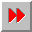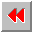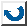EMA-XPS Online

NUMERATOR

```NUMERATOR
=========

syntax: (numerator <ratio>)

==> <numerator>

This function extracts the numerator from a ratio.
The ratio has to be of type integer or ratio. If
the number is an integer then the numerator = <ratio>.

example:       >(numerator 2/3)
2

```EMA-XPS Online